# Ancient and Sacred use of these numbers – 5/7

I was stimulated into realising the importance of my Cube discovery, from information mentioned in books I read recently and a television show I saw on a particular number autistic savant, Daniel Tammet.

Firstly I would like to describe why I believe the number 9 has such underlying importance. As shown in this Ant Cube of number patterns, the number 9 reveals itself as a ‘physical’ structure, totally encompassing the number Cube’s outer shell, with internal intersecting pillars, and wholly different to the other eight numbers 1 to 8. The importance of the three dimensions of the number nine, as well as the Cube coupling numbers, is shown in part by the following:-

With reference to the book ‘Heavens Mirror; Quest for the Lost Civilisation’ by Graham Hancock and Santha Faiia, there are numbers considered of such importance that they are passed down in myth, legend, architecture and religious texts. I would like to point out some very interesting links between these numbers mentioned and the number patterns discovered in the Ant Cube (i.e. the ‘structural’ number 9 and the coupling numbers). At the time of writing this I have not yet made contact with the authors to discuss these links. Also there is no mention that I could find, in their wonderful book, of these links.

Firstly regarding the number 9 being a possible ‘structure’ inherent in the mathematics of our measurement of time and the universe, please note the following extracts from their book i.e.

Page 150. “The great ‘world ages’ of Hindu cosmology… Their respective durations are 1,728,000 years, 1,296,000 years, 864,000 years and 432,000…  (and with respect to Angkor Wat) the key sections of the causeway 1,728 Hat, 1,296 Hat, 864 Hat and 432 Hat – the Yuga lengths scaled down by 1000… passage of time is numerically expressed by the length corresponding to yugas along the west – east axis”.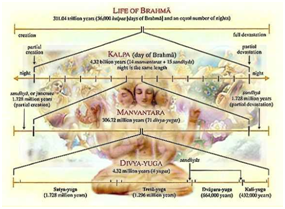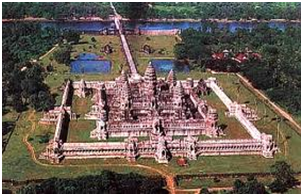Aerial view Angkor Wat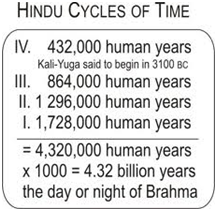My comment: As well as the 4, 3, 2 ratio between these periods of time, when adding the number integers until a single number is reached, each of these ancient number periods add up to the number nine, the ‘structural number’ of our universe:- i.e.

1,728,000 years and 1,728 Hat = 1 + 7 + 2 + 8 (+0 +0 + 0) = 18 = 1 + 8 = 9;

(note coupling numbers 1 + 8 [7 + 2 + 8] = 9)

1,296,000 years and 1,296 Hat = 1 + 2 + 9 + 6 = 18 = 1 + 8 = 9;

(note coupling numbers 3 [1 + 2 + 9] + 6 = 9).

864,000 years and 864 Hat =  8 + 6 + 4 = 18 = 1 + 8 = 9;

(note coupling numbers 8 + 1 [6 + 4 = 1] or 5 [8 + 6] + 4 = 9).

432,000 years and 432 Hat = 4 + 3 + 2 = 9;

(note coupling numbers 4 + 5 [3 + 2], or 7 [4 + 3] + 2 = 9).

The grand total of the ‘World Ages’ of Hindu cosmology = 4,320,000 years. (A reminder that the ratio of the ‘world ages’ is also 4 to 3 to 2 and 4 + 3 + 2 = 9.)

At this point I would like to mention that this number 4,320,000 or its division by 10 = 432,000 or again by 10 = 43,200 is mentioned in many ancient forms such as the following:

• Kali Yuga, the last of the four world ages mentioned above describes 1200 god years of 360 mortal years, which equals 1200 x 360 = 432,000 mortal years.
• The ancient religious Hindu texts, the Rig Veda, has 10,800 stanzas of 40 syllables each, which equals 10,800 x 40 = 432,000 syllables.
• The ratio of the scale of the Great Pyramid to our planet’s circumference of 1: 43,200 has been hinted at from ancient times. Note that the number 43,200 divided by 2 = 21,600 (2 + 1 + 6 = 9), which is the number of nautical miles in the circumference of our planet. The perimeter of the base of the Great Pyramid of Giza, as described further on, is to all intents and purposes, 0.5 nautical miles, which when multiplied by the 43,200 ratio of the Great Pyramid equals the 21,600 nautical miles circumference of our planet. I discuss this in more detail further on.

Continuing with extracts from ‘Heavens Mirror; Quest for the Lost Civilisation’ by Graham Hancock and Santha Faiia,

Page 164. In discussing the pyramid mountain Pnohm Bakheng, north of Angkor Watt, the central sanctuary is surrounded by 108 towers.  “The number 108, one of the most sacred in Hindu and Buddist cosmologies, is the sum of 72 and 36 (i.e 72 + half of 72). As such it is a key component in the sequence of numbers linked to the earth’s axial precession… Great Cycle of 25,920 years, at the rate of one degree every 72 years.”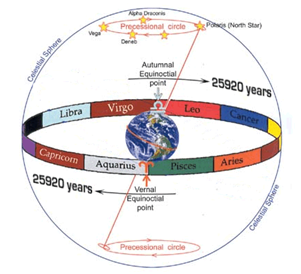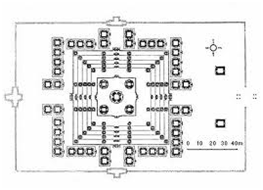Image Great Cycle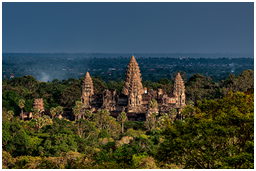My comment: The Great Cycle refers to the axial precessional cycle of our planet: If one extends, as a straight rod, the longitudinal axis of our planet into space, over time this rod slowly moves in a circle. It takes 72 years, approximately, per degree of this 360 degree circle, which means that it takes 72 x 360 years to complete the Great Cycle = 25,920 years. Effectively for an astronomer looking up to the heavens from Earth it would take 72 years for the stars to move just one degree in the sky. For an older civilisation to know about this Great Cycle would be considered highly unlikely without highly sophisticated equipment; and yet it seems some very early civilisations did possess this knowledge.

The sums of the numbers above, each added as per the logic of the number Cube, are as follows;- The sacred numbers  108 = 1 + 0 + 8 = 9; – 72 = 7 + 2 = 9;- 36 = 3 + 6 = 9; –  The Great Сycle of 25,920 years = 2 + 5 + 9 + 2 + 0 = 18 = 9.  The numbers all add up to the structural number 9.

Before bringing in other extracts from the book ‘Heavens Mirror’ I also wish to remind readers of the important ‘coupling’ of numbers revealed in my Cube and how these ‘couplings’ are prevalent in these ancient numbers i.e. the numbers 1 and 8 are of equal pattern but at 90 degrees to one another and can be shown as 18 or 81 or 108 etc. Similarly the numbers 2 and 7 are shown as 72 or 27; 3 and 6 as 36 or 63; 4 and 5 as 54 or 45. Note the numbers in the paragraph above 108, 72 and 36 each reflecting this number ‘coupling’ of 1 with 8, 7 with 2 and 3 with 6. Again I remind the reader that each ‘coupling’ adds up to the structural number 9.

With regard to larger numbers such as 216, optional number couplings may apply. 2 + 1 + 6 =9 and furthermore in terms of number couplings 216 may read as 2 with 7 (1+6) as well as 3 (2 + 1) with 6.

Similarly with large numbers such as noted in the previous page, ‘1296 Hat’, adjacent numbers may be added in any combination, but will still end up with the coupling numbers. i.e. 1296 = coupling numbers

1 with 8 ( 2 + 9 + 6 = 17 = 1 + 7 = 8) or 3 (1 + 2) with 6 (9 + 6 = 15 = 6) or again 3 (1 + 2 + 9 = 12 = 3) with 6.

### Further extract references to ‘Heavens Mirror’

Angkor Watt in Cambodia sits 72 degrees east of the Great Pyramid of Giza, and the overall major building works consists of 72 temple complexes, including Angkor Watt and Angkor Thom.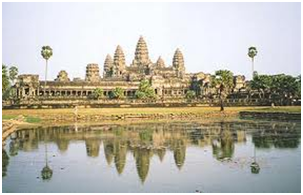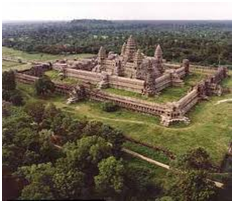Page 184.- regarding the Cambodian ancient temple complex of Angkor Thom where each of the five gates is “surmounted by four serene and gigantic sculpted faces…oriented with high precision towards the four cardinal directions” and “is approached by a bridge, and each … is lined by parallel rows of 54 devas  and 54 asuras – ‘108 per avenue……altogether 540 statues……’a colossal model set up’ of the precessional cycle.”
My comment: 54, 540 and 108 are the number couplings 5 with 4, and 1 with 8 in the Cube and both add up to the ‘structural’ number 9

Page 189 “ ….54 (or 72, or 108, or 216, etc)  … the numerical code by which the cosmic cycle of precession was evoked in ancient cultures.”

My comment: the numbers add up to 9 and are the cube number couplings 5 with 4, 7 with 2, 1 with 8 and 3 (1+2) with 6

Page 190 “there are ‘72 major stone and brick temples and monuments of Angkor’… located 72 degrees of longitude east of the Pyramids of Giza) is, in our view, highly suggestive of an overall plan.”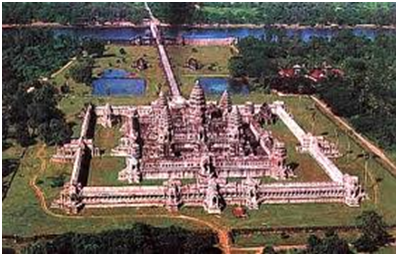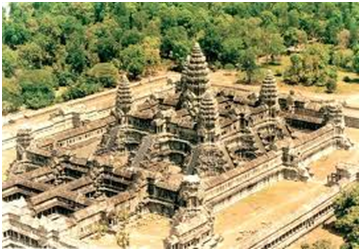My comment: If one takes the centre of the Great Pyramid as 0 degrees longitude (instead of Greenwich, London) then Angkor lies 72 degrees east of the Great Pyramid and has 72 temples (or temple complexes). Significant ancient and Sacred sites lie on degrees longitude which relate directly to the coupling numbers in the Number Cube. 7 and 2 are coupling numbers in this example, adding to 9.

Page 194. At the exact geometric centre of enclosure of Angkor Thom is the Bayon “ the Bayon, 54 massive stone towers, each … is carved with four gigantic faces… making a total of 216 faces”.

My comment: 54 is the Cube number coupling 5 with 4 and adds up to 9. Also 216 faces = 2 + 1 (3) + 6 = 9.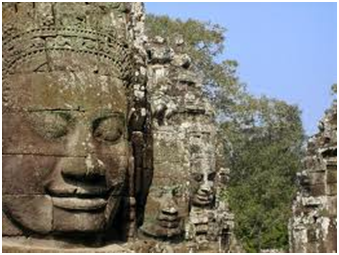Page 201, 203.  “54 degrees of longitude to the east of Angkor… Nan Midol… extensive underwater ruins”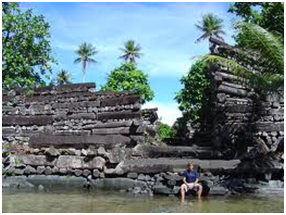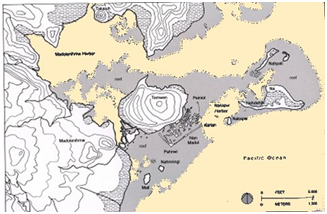My comment: 54 is the cube number coupling of 5 with 4 and adds to 9. Nan Midol is therefore 72 degrees (number coupling 7 with 2) + 54 degrees, east of the Great Pyramid of Giza = 126 degrees east = 1 + 2 + 6 = 9

Page 220. In discussing the changes to the angles of the earth’s obliquity of the ecliptic “ the range of the obliquity cycle is 2 degrees 24 minutes. Each degree is subdivided into 60 arc minutes… a total of 144 arc minutes… total of 8640 arc seconds “.

My comment:  144 adds up to 1 + 4 + 4 = 9, note arc seconds 8 + 6 + 4 + 0 = 18 = 1 + 8 = 9. Again the ‘structural’ number 9 and coupling numbers are inherent in the movement of earth over time. The logic of the coupling numbers with these larger numbers applies as described previously.My comment:  216 adds 2 + 1 + 6 = 9, the structural number I believe, behind the mathematics of our universe. Note that the circumference of Earth is also 21,600 nautical miles. It seems that there is a coincidence in the same number 21,600, applying to both the time required for the minimum to maximum shift of the tilt of the earth’s axis and the number of nautical miles around the earth’s axis. Has anyone else noticed this?  Also I now notice another interesting coincidence! – The number of years required for the tilt of the earth’s axis from maximum back to maximum, minimum back to minimum, which is 43,200 (21,600 x 2) years, is also the ratio in scale of the Great Pyramid to Earth ( i.e. 1 in 43,200. I discuss this ratio further on).Page 220 “If we now divide these 8640 seconds by 40 seconds (the estimated change of obliquitry in a century) we find that 216 centuries- i.e. 21,600 years – is the period required for the tilt of the earth’s axis to shift from its minimum to its maximum value”.

My comment continued; Note that the number 20, or its multiple as 40 (seconds as above), and 60 (arc minutes) above that, not itself divisible by 9, also often plays a role with 9 and the other number couplings in many of these calculations, but with the result still divisible by 9. I mention this now because when looking at the DNA units of life itself, the number 20 (and its multiple) is significant.

The next paragraph in this book ‘Heavens Mirror’ is undoubtedly a co-incidence (?) because it relates to our present point in time, however please note that these co-incidental numbers add back to 9, which may mean that our present time is somehow significant.(As these numbers change they would no longer add to 9) i.e.

“Using the accepted rate of change of 40 arc seconds per century, the earth’s last period of maximum obliquity must have occurred 94.5 centuries ago (3780 divided by 40 = 94.5), i.e. 9450 years before the present”.

(My comment 3780 adds 3 + 7 + 8 + 0 = 18 = 1 + 8 = 9; and also 9450 = 9 + 4 + 5 + 0 = 18 = 1 + 8 = 9. )

Interestingly it is also mentioned that the Hindus and Mayans believed that the New Age started 3100 BC and the Mayan end is 2012 AD.

(My comment: This is a period of 5112 years and 5 + 1 + 1 + 2 = 9. Of course every nine years on the date will again add to 9 so not particularly significant, apart from tying in with the Mayan end of calendar)

Page 221. “..a network of monuments was set up around the world in prehistoric times… co-ordinates of longitude and latitude… through a sequence of ‘precessional numbers’ : 54, 72, 108, 144, 180, 216, etc”

My comment: These numbers expressing degrees longitude all add up to 9 and express the number couplings 1 and 8 (108, 180), 2 and 7 (72), 3 and 6 (216 = 2+1 and 6 = 3 and 6), and 4 and 5 (144 = 1+4 and 4 = 5 and 4). The knowledge of the number couplings, discovered in the Cube highlight that the following, or combinations of the following, many covered above, may further aid in discovering ancient sites of importance from the 0 degree longitude base line of the Giza Pyramids (actually the Great Pyramid) i.e. 18 and 81, 27 and 72, 36 and 63, 45 and 54. Combinations 81 and 27 or 72 and 36 or 63 and 45 = 108; 72 and 45, 63 and 54 = 117; 81 and 54, 72 and 63 = 135; 81 and 63 = 144; 81 and 72 = 153. Also additions such as 108 +108 = 216 or 144 + 72 = 216. In terms of the coupling numbers, the three longitudes up to 180 degrees (half way around the world east or west from the Great Pyramid) that I did not see mentioned in Heaven’s Mirror are 117, 135 and 153; these may reveal important ancient sites yet to be discovered. Similarly longitude mirrored numbers between 180 and 360 degrees or working west from the Great Pyramid.

I need to investigate the relationship, if it exists, of the coupling numbers to latitude also. If a coupling number link to latitude is found to the sites we know of then all of the possible positions for these ancient markers of ancient ‘sacred’ sites could be identified. The reference in old texts that Angkor Watt complex of 72 temples was built over old sacred ‘mounds,’ hints at a very much older positioning of sites around the ancient world, placed on a careful grid of coupling number inter-relationships.

In terms of the latitude of these ancient sacred sites there is a convincing hypothesis in www.Ancient-World-Mysteries.com, by Keith M Hunter and various other authors, such as Jim Alison, that the majority of these ancient sacred sites such as Giza, Angkor, Nazca, Ollantaytambo, Easter Island etc, sit on one Great Circle around earth, tilted in a way that crosses the equator so that an equal half is above and the other half below. When rotating the earth visually along the equator, the Great Circle creates a single great sine wave, with a wave height equal to half the radius of earth. The distance between the sites have a strong ‘golden section’ relationship i.e. 1.618, as does the Great pyramid (length both sides divided by the base length, 712/440 = 1.618).

My comment: If the above hypotheses are true then the coupling numbers of the number Cube are reflected only in the longitude positioning of these mysterious ancient sites, and the latitude is determined by their location on the Great Circle. As an aside, does another such Great Circle exist, picking up other ‘sacred’ sites?

I believe that there is an exception to the greatest significant ancient sites being located on the Great Circle, and that is the locations of the legendary ‘Halls of Records’ (i.e. sites containing secrets of the universe, hidden by the ‘Gods,’ and undiscoverable by chance, according to the Egyptian ancient texts); they would otherwise be too easy to find, in my opinion. According to the author Ralph Ellis, and as discussed on the next page, the one possible Hall of Records site may exist at K2. The dead centre of this site has the number coupling result in both longitude and latitude, i.e 45 degrees east of the Great Pyramid and 36 degrees north of the equator, both coordinates separately and together adding to the structural number 9. To my sensibilities and logic, this rings an important bell, and points to a site of great importance in terms of the Cube number couplings.